Full Report for Catchup (Ketchup 6.0) by Nick Bentley

# Full Report for Catchup (Ketchup 6.0) by Nick Bentley

Play one or two pieces per turn, may play a third if you are behind. Largest group wins when the board is full.

Generated at 05/10/2020, 23:09 from 159802 logged games.

## Rules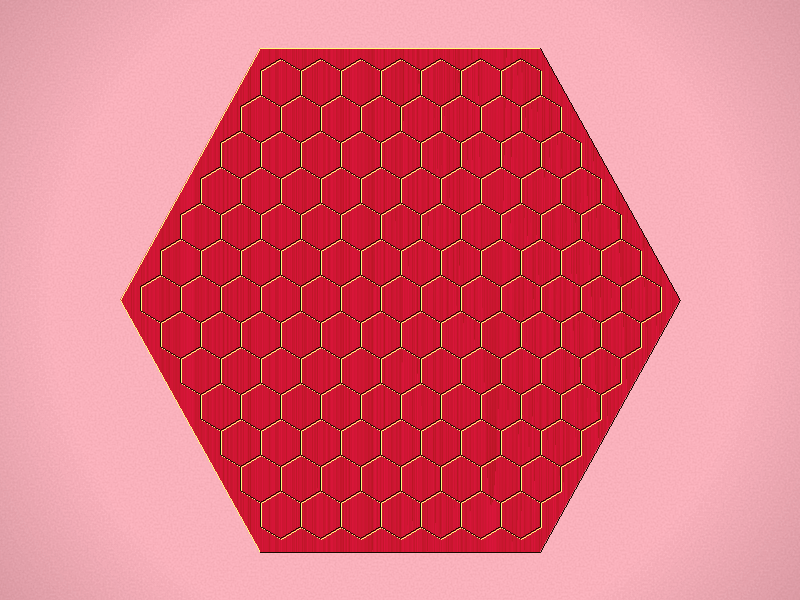Representative game (in the sense of being of mean length). Wherever you see the 'representative game' referred to in later sections, this is it!

Prep

Before the game starts, set one white pawn and one black pawn on the "1" space on the scoring track. These will be moved along the scoring track as the game goes along to mark the size of each players largest group.

Rules

1. One player owns the white stones and the other owns the black. White begins by dropping 1 stone.
2. From then on, starting with Black, each player must drop 1 or 2 stones on her turn.
3. If you increase the size of your largest group, move your pawn to the corresponding space on the scoring track. If you move your pawn up to or past your opponents pawn on the scoring track, your opponent may drop up to 3 stones on her next turn.
4. The game ends when the board is full. The player with the largest group wins. If the players largest groups are the same size, compare their second-largest groups, and so on, until you come to a pair which arent the same size. Whoever owns the larger of the two wins.

## Miscellaneous

Play: Combinatorial

Family: Combinatorial 2017

Mechanism(s): Group,Strict Placement

Components: Board

## BGG Stats

BGG Entry Catchup (Ketchup 6.0) null null null null null null

## Kolomogorov Complexity Analysis

 Size (bytes) 32861 10293 3.19

Ai Ai calculates the size of the implementation, and compares it to the Ai Ai implementation of the simplest possible game (which just fills the board). Note that this estimate may include some graphics and heuristics code as well as the game logic. See the wikipedia entry for more details.

## Playout Complexity Estimate

Playouts per second 67059.19 (14.91µs/playout) 1636125.65 (0.61µs/playout) 24.40

Tavener complexity: the heat generated by playing every possible instance of a game with a perfectly efficient programme. Since this is not possible to calculate, Ai Ai calculates the number of random playouts per second and compares it to the fastest non-trivial Ai Ai game (Connect 4). This ratio gives a practical indication of how complex the game is. Combine this with the computational state space, and you can get an idea of how strong the default (MCTS-based) AI will be.

## Playout/Search Speed

LabelIts/sSDNodes/sSDGame lengthSD
Random playout72,9058239,459,585106,8061302
search.UCB71,4099711281
search.UCT70,8259481291

Random: 10 second warmup for the hotspot compiler. 100 trials of 1000ms each.

Other: 100 playouts, means calculated over the first 5 moves only to avoid distortion due to speedup at end of game.

## Mirroring Strategies

Rotation (Half turn) lost each game as expected.
Reflection (X axis) lost each game as expected.
Reflection (Y axis) lost each game as expected.
Copy last move lost each game as expected.

Mirroring strategies attempt to copy the previous move. On first move, they will attempt to play in the centre. If neither of these are possible, they will pick a random move. Each entry represents a different form of copying; direct copy, reflection in either the X or Y axis, half-turn rotation.

## Win % By Player (Bias)

1: White win % 2: Black win % 49.95±0.25 Includes draws = 50% 50.05±0.25 Includes draws = 50% 0.00 Percentage of games where all players draw. 100.00 Percentage of games with a single winner. 159802 Quantity of logged games played

Note: that win/loss statistics may vary depending on thinking time (horizon effect, etc.), bad heuristics, bugs, and other factors, so should be taken with a pinch of salt. (Given perfect play, any game of pure skill will always end in the same result.)

Note: Ai Ai differentiates between states where all players draw or win or lose; this is mostly to support cooperative games.

## UCT Skill Chains

MatchAIStrong WinsDrawsStrong Losses#GamesStrong Scorep1 Win%Draw%p2 Win%Game Length
0Random
1UCT (its=2)63103539840.6108 <= 0.6413 <= 0.670650.100.0049.90129.75
2
UCT (its=3)
549
0
451
1000
0.5180 <= 0.5490 <= 0.5796
50.30
0.00
49.70
129.69
3
UCT (its=4)
580
0
420
1000
0.5492 <= 0.5800 <= 0.6102
51.40
0.00
48.60
129.65
4
UCT (its=5)
592
0
408
1000
0.5612 <= 0.5920 <= 0.6221
48.40
0.00
51.60
129.51
5
UCT (its=6)
630
0
370
1000
0.5996 <= 0.6300 <= 0.6594
47.80
0.00
52.20
129.59
6UCT (its=7)63103499800.6134 <= 0.6439 <= 0.673248.880.0051.12129.59
7
UCT (its=8)
525
0
475
1000
0.4940 <= 0.5250 <= 0.5558
51.30
0.00
48.70
129.48
8
UCT (its=9)
547
0
453
1000
0.5160 <= 0.5470 <= 0.5776
50.70
0.00
49.30
129.50
9
UCT (its=10)
538
0
462
1000
0.5070 <= 0.5380 <= 0.5687
46.40
0.00
53.60
129.49
10
UCT (its=11)
533
0
467
1000
0.5020 <= 0.5330 <= 0.5637
51.90
0.00
48.10
129.45
11
UCT (its=12)
580
0
420
1000
0.5492 <= 0.5800 <= 0.6102
51.20
0.00
48.80
129.47
12
UCT (its=13)
581
0
419
1000
0.5502 <= 0.5810 <= 0.6112
47.10
0.00
52.90
129.38
13
UCT (its=14)
602
0
398
1000
0.5713 <= 0.6020 <= 0.6319
47.00
0.00
53.00
129.39
14
UCT (its=15)
614
0
386
1000
0.5834 <= 0.6140 <= 0.6437
50.20
0.00
49.80
129.44
15
UCT (its=16)
621
0
379
1000
0.5905 <= 0.6210 <= 0.6506
49.70
0.00
50.30
129.52
16UCT (its=17)63103649950.6038 <= 0.6342 <= 0.663551.960.0048.04129.42
17
UCT (its=18)
558
0
442
1000
0.5271 <= 0.5580 <= 0.5885
50.80
0.00
49.20
129.39
18
UCT (its=19)
551
0
449
1000
0.5200 <= 0.5510 <= 0.5816
49.50
0.00
50.50
129.29
19
UCT (its=20)
540
0
460
1000
0.5090 <= 0.5400 <= 0.5707
49.60
0.00
50.40
129.27
20
UCT (its=21)
565
0
435
1000
0.5341 <= 0.5650 <= 0.5954
51.10
0.00
48.90
129.38
21
UCT (its=22)
588
0
412
1000
0.5572 <= 0.5880 <= 0.6181
48.20
0.00
51.80
129.35
22
UCT (its=23)
587
0
413
1000
0.5562 <= 0.5870 <= 0.6171
50.30
0.00
49.70
129.33
23
UCT (its=24)
622
0
378
1000
0.5915 <= 0.6220 <= 0.6515
51.80
0.00
48.20
129.37
24UCT (its=25)63103539840.6108 <= 0.6413 <= 0.670650.100.0049.90129.35
25
UCT (its=26)
522
0
478
1000
0.4910 <= 0.5220 <= 0.5528
52.60
0.00
47.40
129.29
26
UCT (its=27)
529
0
471
1000
0.4980 <= 0.5290 <= 0.5598
49.30
0.00
50.70
129.29
27
UCT (its=28)
556
0
444
1000
0.5250 <= 0.5560 <= 0.5865
48.60
0.00
51.40
129.34
28
UCT (its=29)
580
0
420
1000
0.5492 <= 0.5800 <= 0.6102
52.00
0.00
48.00
129.23
29
UCT (its=30)
561
0
439
1000
0.5301 <= 0.5610 <= 0.5915
49.10
0.00
50.90
129.28
30
UCT (its=31)
609
0
391
1000
0.5784 <= 0.6090 <= 0.6388
49.90
0.00
50.10
129.27
31
UCT (its=32)
588
0
412
1000
0.5572 <= 0.5880 <= 0.6181
52.20
0.00
47.80
129.33
32
UCT (its=33)
606
0
394
1000
0.5754 <= 0.6060 <= 0.6358
49.20
0.00
50.80
129.39
33
UCT (its=34)
614
0
386
1000
0.5834 <= 0.6140 <= 0.6437
52.20
0.00
47.80
129.30
34UCT (its=35)63103269570.6287 <= 0.6594 <= 0.688749.530.0050.47129.28
35
UCT (its=36)
510
0
490
1000
0.4790 <= 0.5100 <= 0.5409
51.40
0.00
48.60
129.25
36
UCT (its=37)
538
0
462
1000
0.5070 <= 0.5380 <= 0.5687
51.60
0.00
48.40
129.23
37
UCT (its=38)
536
0
464
1000
0.5050 <= 0.5360 <= 0.5667
48.20
0.00
51.80
129.17
38
UCT (its=39)
565
0
435
1000
0.5341 <= 0.5650 <= 0.5954
52.30
0.00
47.70
129.27
39
UCT (its=40)
544
0
456
1000
0.5130 <= 0.5440 <= 0.5746
51.40
0.00
48.60
129.19
40
UCT (its=41)
571
0
429
1000
0.5401 <= 0.5710 <= 0.6013
47.30
0.00
52.70
129.14
41
UCT (its=42)
586
0
414
1000
0.5552 <= 0.5860 <= 0.6161
50.00
0.00
50.00
129.16
42
UCT (its=43)
618
0
382
1000
0.5875 <= 0.6180 <= 0.6476
50.80
0.00
49.20
129.13
43
UCT (its=44)
616
0
384
1000
0.5855 <= 0.6160 <= 0.6456
50.00
0.00
50.00
129.16
44
UCT (its=45)
615
0
385
1000
0.5845 <= 0.6150 <= 0.6447
48.30
0.00
51.70
129.20
45UCT (its=46)63103509810.6127 <= 0.6432 <= 0.672651.170.0048.83129.30
46
UCT (its=47)
499
0
501
1000
0.4681 <= 0.4990 <= 0.5299
52.90
0.00
47.10
129.12
47
UCT (its=48)
514
0
486
1000
0.4830 <= 0.5140 <= 0.5449
48.40
0.00
51.60
129.22
48
UCT (its=49)
526
0
474
1000
0.4950 <= 0.5260 <= 0.5568
52.20
0.00
47.80
129.06
49
UCT (its=50)
542
0
458
1000
0.5110 <= 0.5420 <= 0.5727
48.60
0.00
51.40
129.24
50
UCT (its=51)
529
0
471
1000
0.4980 <= 0.5290 <= 0.5598
48.70
0.00
51.30
129.16
51
UCT (its=52)
569
0
431
1000
0.5381 <= 0.5690 <= 0.5994
49.30
0.00
50.70
129.20
52
UCT (its=53)
543
0
457
1000
0.5120 <= 0.5430 <= 0.5737
51.50
0.00
48.50
129.05
53
UCT (its=54)
603
0
397
1000
0.5723 <= 0.6030 <= 0.6329
50.10
0.00
49.90
129.21
54
UCT (its=55)
592
0
408
1000
0.5612 <= 0.5920 <= 0.6221
51.00
0.00
49.00
129.12
55
UCT (its=56)
596
0
404
1000
0.5653 <= 0.5960 <= 0.6260
49.40
0.00
50.60
129.22
56
UCT (its=57)
599
0
401
1000
0.5683 <= 0.5990 <= 0.6289
50.10
0.00
49.90
129.17
57
UCT (its=58)
617
0
383
1000
0.5865 <= 0.6170 <= 0.6466
48.10
0.00
51.90
129.05
58
UCT (its=59)
590
0
410
1000
0.5592 <= 0.5900 <= 0.6201
51.20
0.00
48.80
129.08
59
UCT (its=60)
598
0
402
1000
0.5673 <= 0.5980 <= 0.6280
50.80
0.00
49.20
129.30
60UCT (its=61)63103289590.6274 <= 0.6580 <= 0.687349.320.0050.68129.24
61
UCT (its=62)
499
0
501
1000
0.4681 <= 0.4990 <= 0.5299
50.30
0.00
49.70
129.08
62
UCT (its=63)
539
0
461
1000
0.5080 <= 0.5390 <= 0.5697
49.70
0.00
50.30
129.10
63
UCT (its=64)
514
0
486
1000
0.4830 <= 0.5140 <= 0.5449
46.80
0.00
53.20
129.19
64
UCT (its=65)
550
0
450
1000
0.5190 <= 0.5500 <= 0.5806
52.20
0.00
47.80
129.06
65
UCT (its=66)
540
0
460
1000
0.5090 <= 0.5400 <= 0.5707
51.80
0.00
48.20
129.13
66
UCT (its=67)
572
0
428
1000
0.5411 <= 0.5720 <= 0.6023
47.80
0.00
52.20
129.14
67
UCT (its=68)
539
0
461
1000
0.5080 <= 0.5390 <= 0.5697
49.70
0.00
50.30
129.06
68
UCT (its=69)
561
0
439
1000
0.5301 <= 0.5610 <= 0.5915
51.90
0.00
48.10
129.08
69
UCT (its=70)
557
0
443
1000
0.5261 <= 0.5570 <= 0.5875
50.10
0.00
49.90
129.09
70
UCT (its=71)
570
0
430
1000
0.5391 <= 0.5700 <= 0.6004
47.60
0.00
52.40
129.00
71
UCT (its=72)
588
0
412
1000
0.5572 <= 0.5880 <= 0.6181
49.20
0.00
50.80
129.14
72
UCT (its=73)
589
0
411
1000
0.5582 <= 0.5890 <= 0.6191
50.70
0.00
49.30
129.15
73
UCT (its=74)
590
0
410
1000
0.5592 <= 0.5900 <= 0.6201
51.20
0.00
48.80
129.05
74
UCT (its=75)
601
0
399
1000
0.5703 <= 0.6010 <= 0.6309
48.90
0.00
51.10
129.11
75
UCT (its=76)
586
0
414
1000
0.5552 <= 0.5860 <= 0.6161
50.60
0.00
49.40
129.15
76
UCT (its=77)
597
0
403
1000
0.5663 <= 0.5970 <= 0.6270
52.50
0.00
47.50
129.13
77
UCT (its=78)
613
0
387
1000
0.5824 <= 0.6130 <= 0.6427
48.30
0.00
51.70
129.05
78
UCT (its=79)
625
0
375
1000
0.5946 <= 0.6250 <= 0.6545
49.10
0.00
50.90
129.05
79UCT (its=80)63103319620.6253 <= 0.6559 <= 0.685349.270.0050.73129.13
80
UCT (its=81)
475
0
525
1000
0.4442 <= 0.4750 <= 0.5060
50.70
0.00
49.30
129.14
81
UCT (its=82)
519
0
481
1000
0.4880 <= 0.5190 <= 0.5498
54.50
0.00
45.50
129.13
82
UCT (its=82)
483
0
517
1000
0.4522 <= 0.4830 <= 0.5140
52.50
0.00
47.50
129.00

Search for levels ended: time limit reached.

Level of Play: Strong beats Weak 60% of the time (lower bound with 95% confidence).

Draw%, p1 win% and game length may give some indication of trends as AI strength increases.

## 1st Player Win Ratios by Playing Strength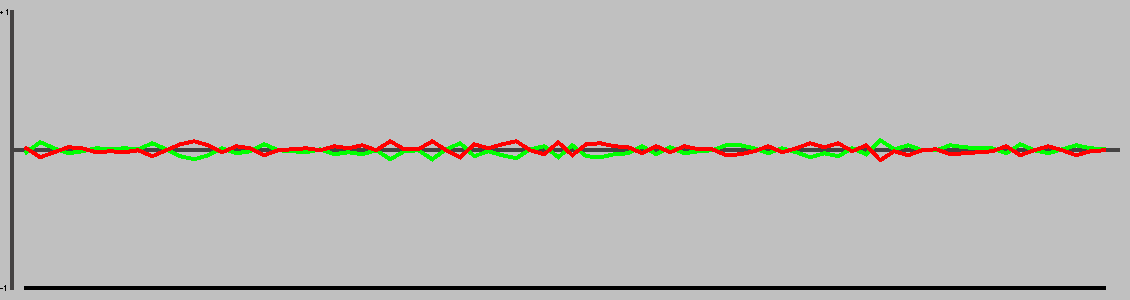This chart shows the win(green)/draw(black)/loss(red) percentages, as UCT play strength increases. Note that for most games, the top playing strength show here will be distinctly below human standard.

## Complexity

 Samples 159802 Quantity of logged games played

Computational complexity (where present) is an estimate of the game tree reachable through actual play. For each game in turn, Ai Ai marks the positions reached in a hashtable, then counts the number of new moves added to the table. Once all moves are applied, it treats this sequence as a geometric progression and calculates the sum as n-> infinity.

## Change in Material Per Turn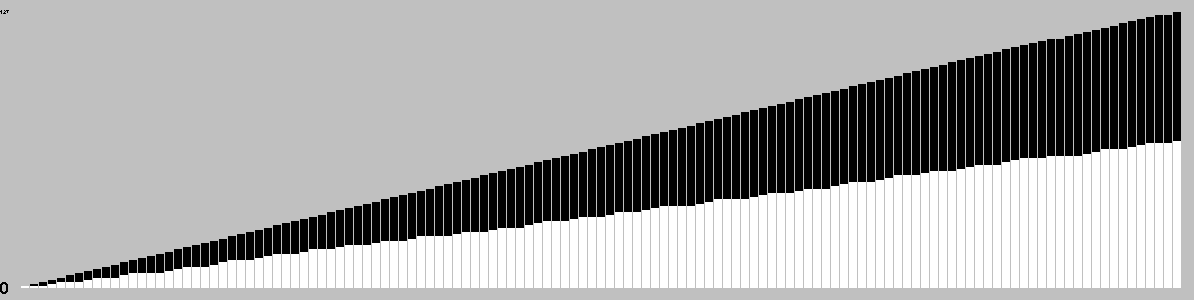This chart is based on a single representative* playout, and gives a feel for the change in material over the course of a game. (* Representative in the sense that it is close to the mean length.)

## Actions/turn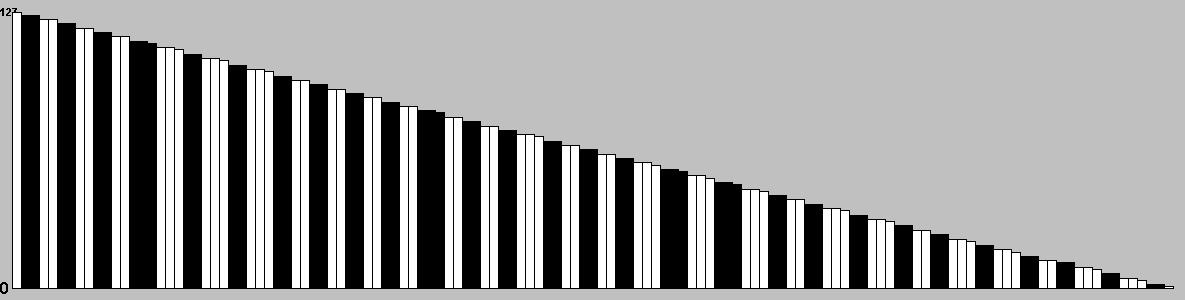Table: branching factor per turn, based on a single representative* game. (* Representative in the sense that it is close to the mean game length.)

## Action Types per Turn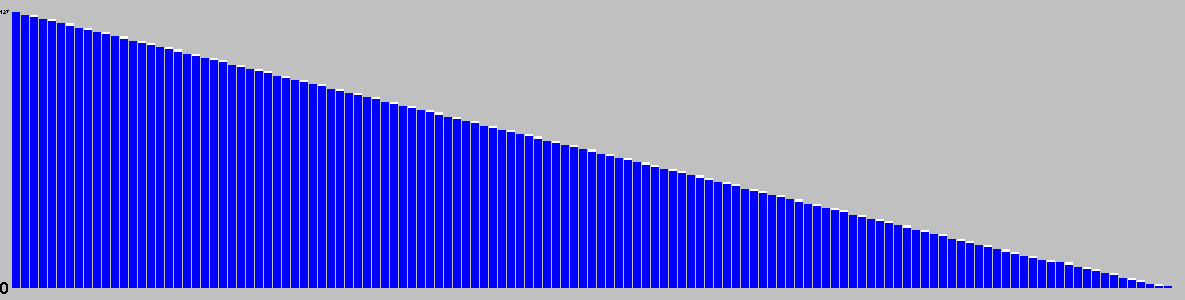This chart is based on a single representative* game, and gives a feel for the types of moves available throughout that game. (* Representative in the sense that it is close to the mean game length.)

Red: removal, Black: move, Blue: Add, Grey: pass, Purple: swap sides, Brown: other.

## Trajectory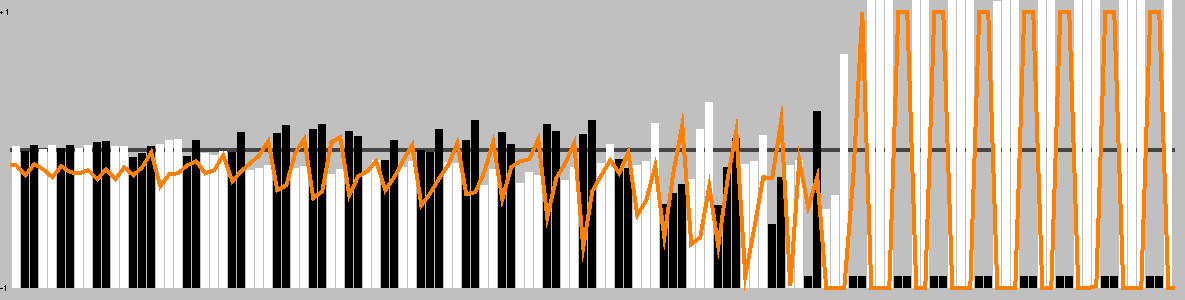This chart shows the best move value with respect to the active player; the orange line represents the value of doing nothing (null move).

The lead changed on 24% of the game turns. Ai Ai found 4 critical turns (turns with only one good option).

## Position Heatmap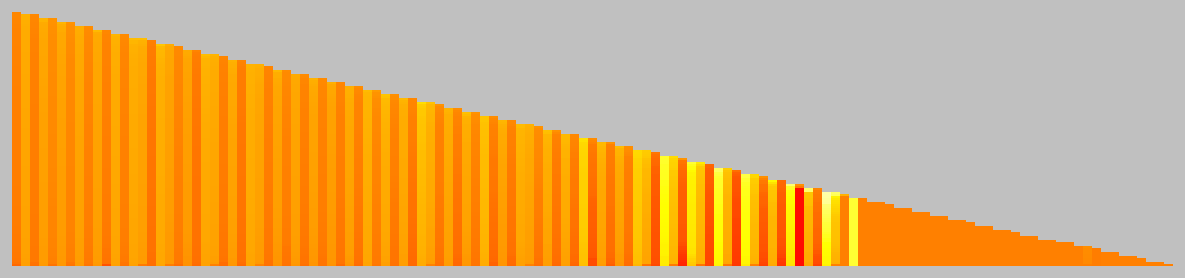This chart shows the relative temperature of all moves each turn. Colour range: black (worst), red, orange(even), yellow, white(best).

## Good/Effective moves

 Distinct actions null Number of distinct moves (e.g. "e4") regardless of position in game tree
MeasureAll playersPlayer 1Player 2
Mean % of effective moves59.7260.4558.88
Mean no. of effective moves33.1432.9033.42
Effective game space10^140.5410^75.3010^65.24
Mean % of good moves47.9441.9954.78
Mean no. of good moves32.2535.7728.20
Good move game space10^119.5410^62.1910^57.36

These figures were calculated over a single game.

An effective move is one with score 0.1 of the best move (including the best move). -1 (loss) <= score <= 1 (win)

A good move has a score > 0. Note that when there are no good moves, an multiplier of 1 is used for the game space calculation.

## Quality Measures

MeasureValueDescription
Hot turns75.97%A hot turn is one where making a move is better than doing nothing.
Momentum22.48%% of turns where a player improved their score.
Correction39.53%% of turns where the score headed back towards equality.
Depth2.40%Difference in evaluation between a short and long search.
Drama0.92%How much the winner was behind before their final victory.
Foulup Factor51.16%Moves that looked better than the best move after a short search.
Surprising turns1.55%Turns that looked bad after a short search, but good after a long one.
Last lead change72.09%Distance through game when the lead changed for the last time.
Decisiveness7.75%Distance from the result being known to the end of the game.

These figures were calculated over a single representative* game, and based on the measures of quality described in "Automatic Generation and Evaluation of Recombination Games" (Cameron Browne, 2007). (* Representative, in the sense that it is close to the mean game length.)

## Openings

MovesAnimation
g2,f9,e3g2,f9,Passc5,g6,Pass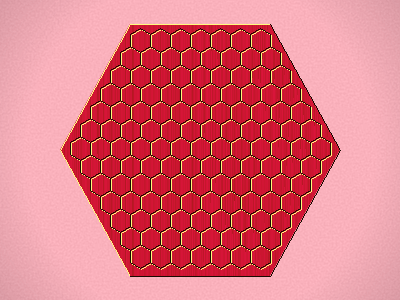f6,j10,Pass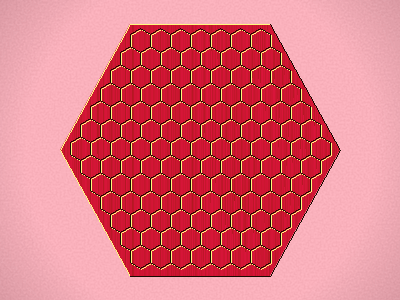e8,d7,m4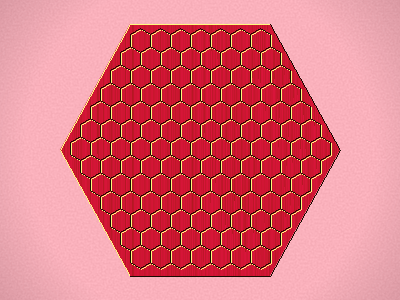f8,f2,e3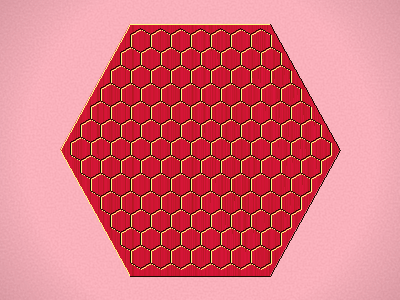k8,f8,Passb9,l6,Passi9,f11,Pass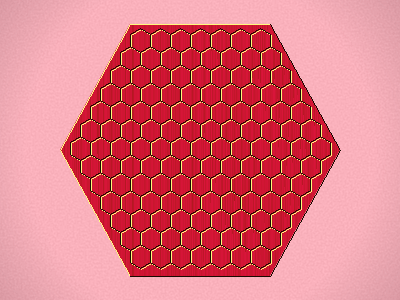d11,m7,h4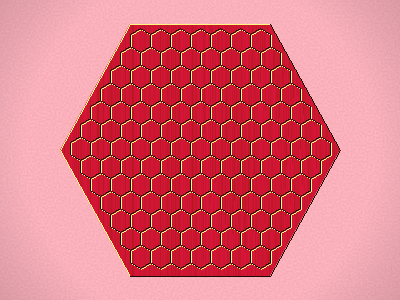h3,d9,Pass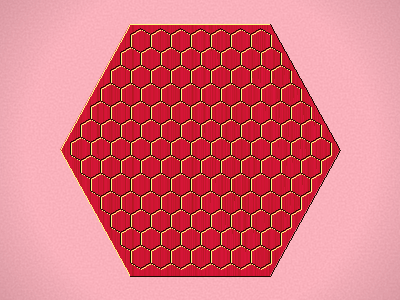h3,d10,Pass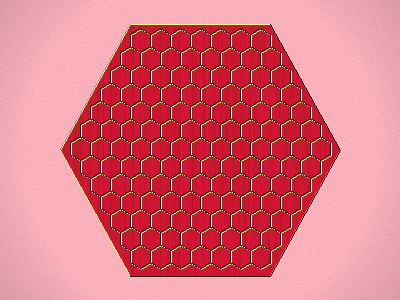## Opening Heatmap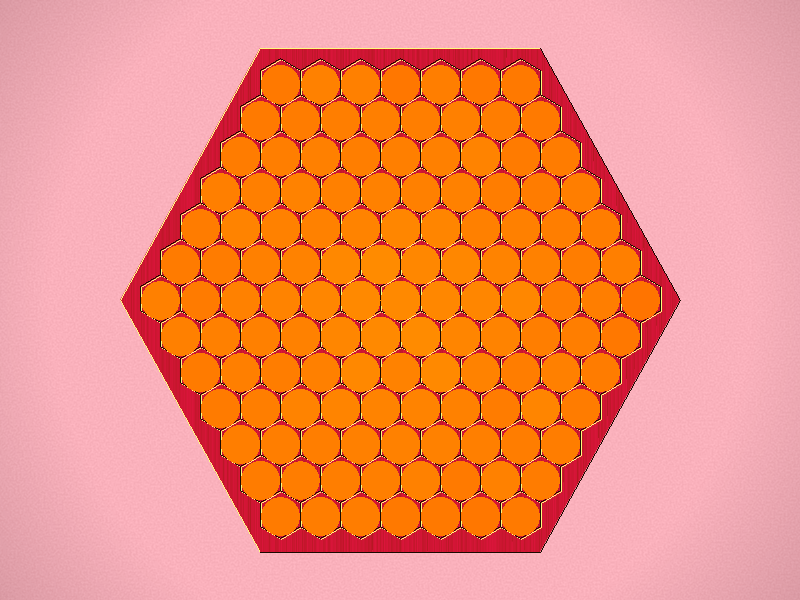Colour shows the success ratio of this play over the first 10moves; black < red < yellow < white.

Size shows the frequency this move is played.

## Unique Positions Reachable at Depth

0123
1127161291016254

Note: most games do not take board rotation and reflection into consideration.
Multi-part turns could be treated as the same or different depth depending on the implementation.
Counts to depth N include all moves reachable at lower depths.
Inaccuracies may also exist due to hash collisions, but Ai Ai uses 64-bit hashes so these will be a very small fraction of a percentage point.

## Shortest Game(s)

No solutions found to depth 3.